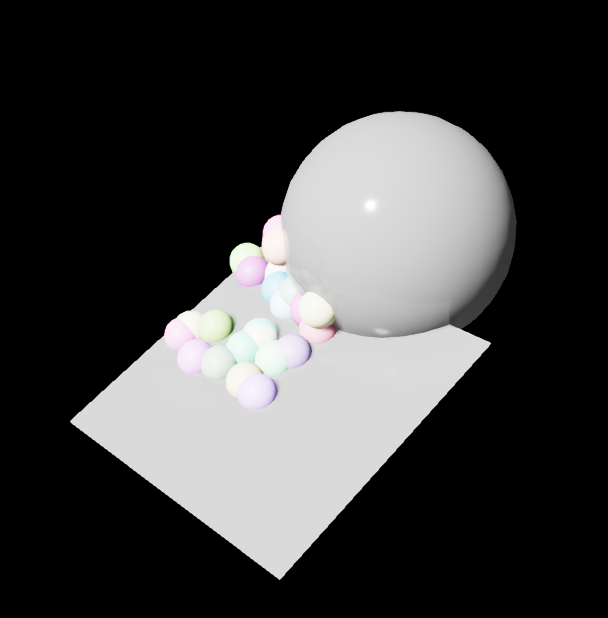# Scatter Examples

The goal of these examples are to provide common use cases for scattering in Omniverse Replicator.

Below are a few demonstrations of scatter applied within a scene using Replicator APIs.

## Learning Objectives

Through this page you will see some highlighted scattering examples and how to use them.

However, for all information about replicator camera use consult the API documentation .

## Scattering objects in a surface

In this example we show you how to scatter objects in a 2D surface using the function `randomizer.scatter_2d()`.

```import omni.graph.core as og
import omni.replicator.core as rep

# create a plane to sample on
plane_samp = rep.create.plane(scale=4, rotation=(20, 0, 0))

def randomize_spheres():
# create small spheres to sample inside the plane
spheres = rep.create.sphere(scale=0.4, count=30)

# randomize
with spheres:
rep.randomizer.scatter_2d(plane_samp)
# Add color to small spheres
rep.randomizer.color(colors=rep.distribution.uniform((0.2, 0.2, 0.2), (1, 1, 1)))
return spheres.node

rep.randomizer.register(randomize_spheres)

with rep.trigger.on_frame(num_frames=10):
rep.randomizer.randomize_spheres()
```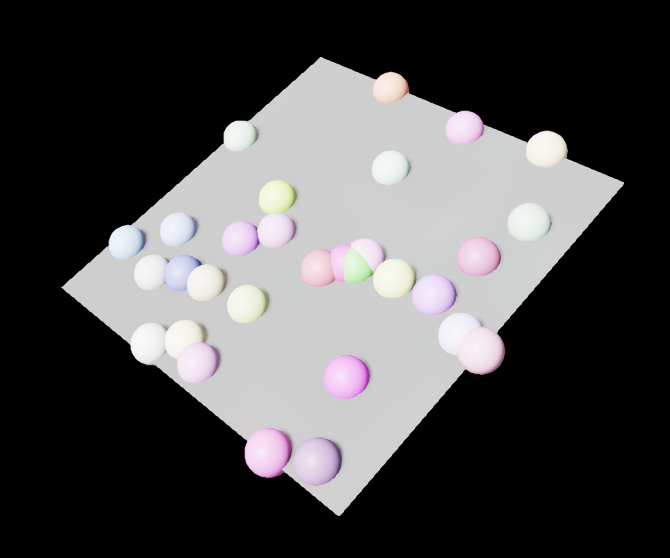The spheres are scattered on the plane, but you should notice that they will intersect with each other.

## Scatter 2D with collisions

Altering the code above, we can add `check_for_collisions=True` to ensure that none of the spheres overlap.

```import omni.graph.core as og
import omni.replicator.core as rep

# create a plane to sample on
plane_samp = rep.create.plane(scale=4, rotation=(20, 0, 0))

def randomize_spheres():
# create small spheres to sample inside the plane
spheres = rep.create.sphere(scale=0.4, count=30)

# randomize
with spheres:
rep.randomizer.scatter_2d(plane_samp, check_for_collisions=True)
# Add color to small spheres
rep.randomizer.color(colors=rep.distribution.uniform((0.2, 0.2, 0.2), (1, 1, 1)))
return spheres.node

rep.randomizer.register(randomize_spheres)

with rep.trigger.on_frame(num_frames=10):
rep.randomizer.randomize_spheres()
```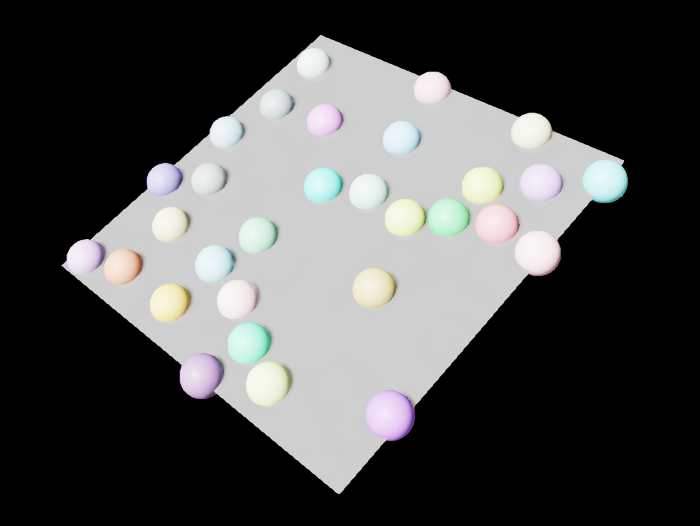The spheres are now scattered without intersecting with each other.

Note

An error will be raised if no collision solution can be found within a reasonable amount of time (for instance: having too many objects)

## Multi-Surface Scatter 2D

In this example, the Scatter 2D node can be used for sampling on multiple surfaces.

We create a second surface, a large sphere, and sample 40 small spheres distributed across both surfaces.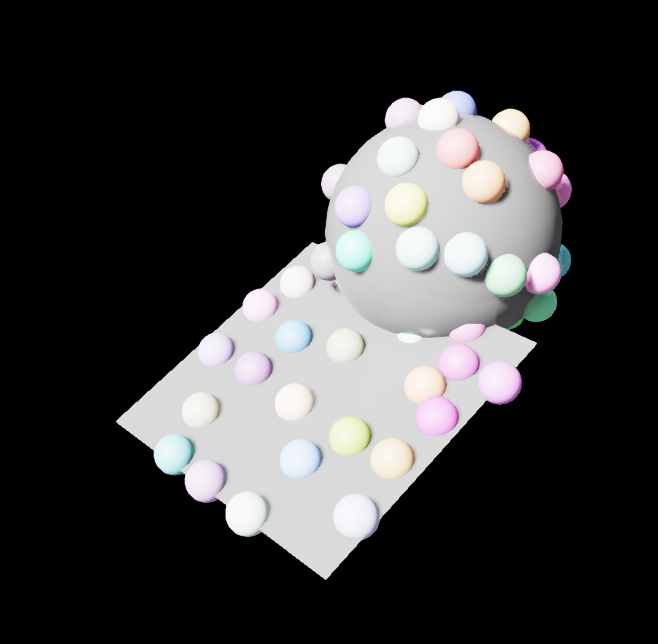```import omni.replicator.core as rep

# A light to see
distance_light = rep.create.light(rotation=(-45, 0, 0), light_type="distant")

# Create a plane to sample on
plane_samp = rep.create.plane(scale=3, rotation=(20, 0, 0))

# Create a larger sphere to sample on the surface of
sphere_samp = rep.create.sphere(scale=2.4, position=(0, 100, -180))

def randomize_spheres():
# create small spheres to sample inside the plane
spheres = rep.create.sphere(scale=0.4, count=40)

# scatter small spheres
with spheres:
rep.randomizer.scatter_2d(surface_prims=[plane_samp, sphere_samp], check_for_collisions=True)
# Add color to small spheres
rep.randomizer.color(colors=rep.distribution.uniform((0.2, 0.2, 0.2), (1, 1, 1)))
return spheres.node

rep.randomizer.register(randomize_spheres)

with rep.trigger.on_frame(num_frames=10):
rep.randomizer.randomize_spheres()
```

## Scatter 2D avoiding objects

In this example we show the Scatter 2D node can allow for collision checking with other prims besides the ones created for sampling.

We create a large cylinder and check for collisions by adding it to the parameter list `no_coll_prims`.

The scattered spheres will now avoid placement where the cylinder is located. Multiple extra prims can be added for collision checking with this parameter.

```import omni.replicator.core as rep

# A light to see
distance_light = rep.create.light(rotation=(-45, 0, 0), light_type="distant")

# Create a plane to sample on
plane_samp = rep.create.plane(scale=3, rotation=(20, 0, 0))

# Create a larger sphere to sample on the surface of
sphere_samp = rep.create.sphere(scale=2.4, position=(0, 100, -180))

# Create a larger cylinder we do not want to collide with
cylinder = rep.create.cylinder(
semantics=[("class", "cylinder")], scale=(2, 1, 2))

def randomize_spheres():
# create small spheres to sample inside the plane
spheres = rep.create.sphere(scale=0.4, count=60)

# scatter small spheres
with spheres:
rep.randomizer.scatter_2d(
surface_prims=[plane_samp, sphere_samp], no_coll_prims=[cylinder], check_for_collisions=True
)
# Add color to small spheres
rep.randomizer.color(colors=rep.distribution.uniform((0.2, 0.2, 0.2), (1, 1, 1)))
return spheres.node

rep.randomizer.register(randomize_spheres)

with rep.trigger.on_frame(num_frames=10):
rep.randomizer.randomize_spheres()
```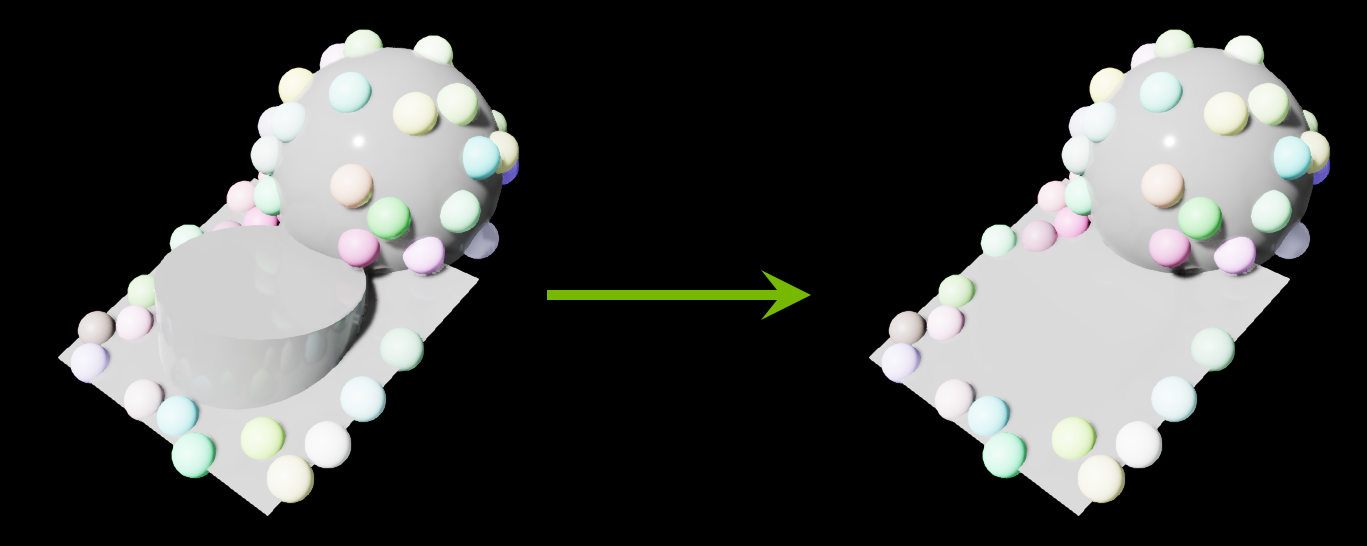## Scatter 2D with limits

In this example, we show that the Scatter 2D node can take in extra parameters for the `min_samp` and `max_samp` sampling bounds.

For example, you might want to stop sampling for everything beyond a certain value, i.e. `y > 100`.

To do this, specify `min_samp=(X, Y, Z)` and/or `max_samp=(X, Y, Z)` in the Scatter 2D node parameters. The `X,Y,Z` values are all in world-space units.

```import omni.replicator.core as rep

# A light to see
distance_light = rep.create.light(rotation=(-45, 0, 0), light_type="distant")

# Create a plane to sample on
plane_samp = rep.create.plane(scale=3, rotation=(20, 0, 0))

# Create a larger sphere to sample on the surface of
sphere_samp = rep.create.sphere(scale=2.4, position=(0, 100, -180))

def randomize_spheres():
# create small spheres to sample inside the plane
spheres = rep.create.sphere(scale=0.4, count=60)

# scatter small spheres
with spheres:
rep.randomizer.scatter_2d(
[plane_samp, sphere_samp],
min_samp=(None, None, None),
# Small spheres will not go beyond 0 in X, 110 in Y, 30 in Z world space
max_samp=(0, 110, 30),
check_for_collisions=False,
)
rep.randomizer.color(colors=rep.distribution.uniform((0.2, 0.2, 0.2), (1, 1, 1)))
return spheres.node

rep.randomizer.register(randomize_spheres)

with rep.trigger.on_frame(num_frames=10):
rep.randomizer.randomize_spheres()
```